## Fundamental Theorems of Calculus

The first fundamental theorem of calculus states that, ifis Continuous on the Closed Intervaland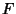is the Antiderivative (Indefinite Integral) ofon, then(1)

The second fundamental theorem of calculus letsbe Continuous on an Open Intervaland letsbe any point in. Ifis defined by(2)

then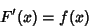(3)

at each point in.

The complex fundamental theorem of calculus states that if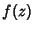has a Continuous Antiderivative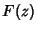in a regioncontaining a parameterized curvefor, then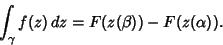(4)